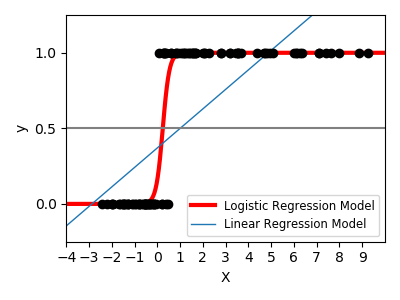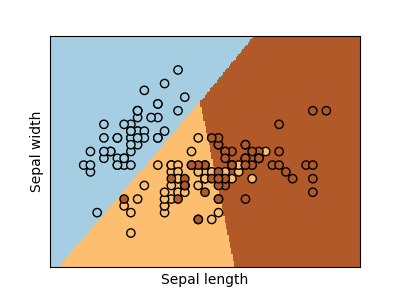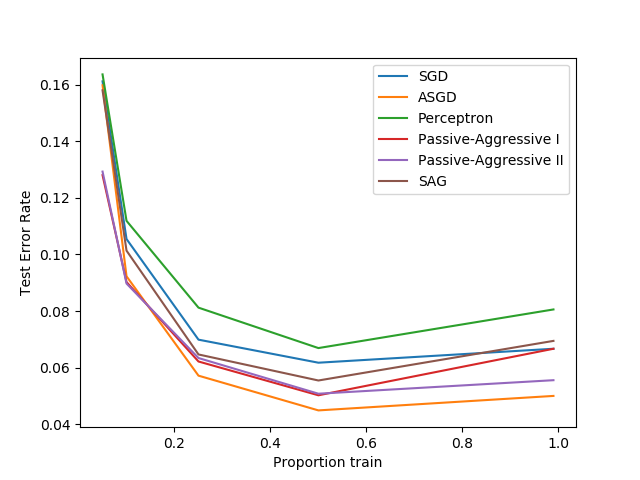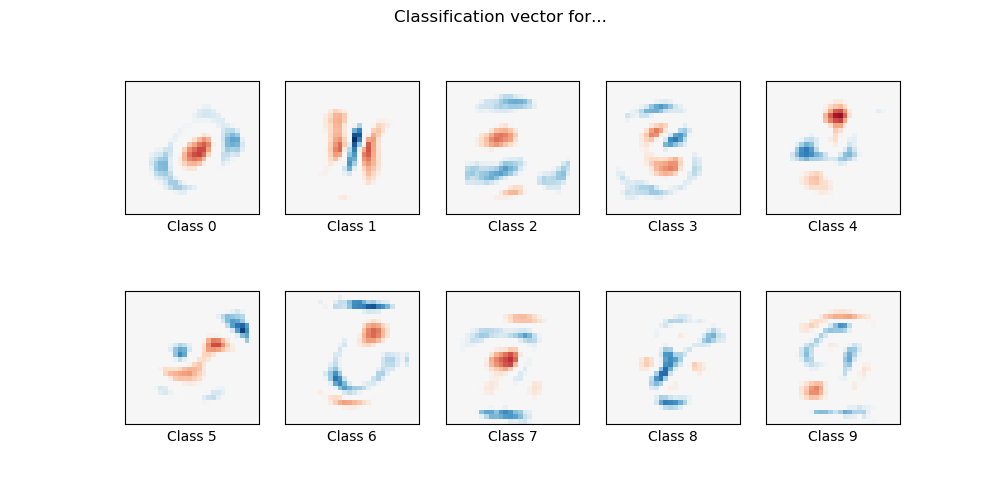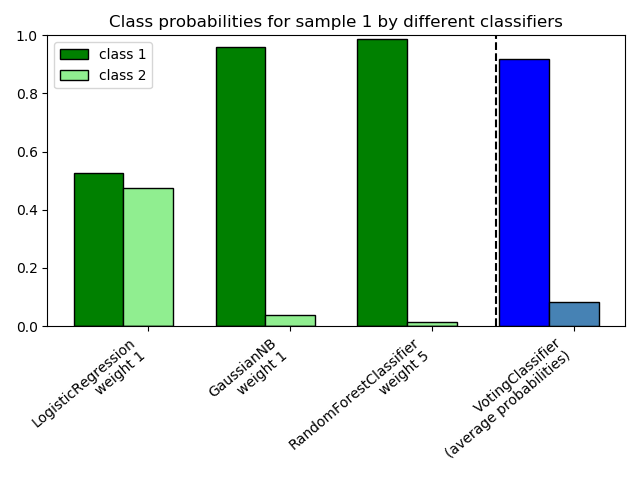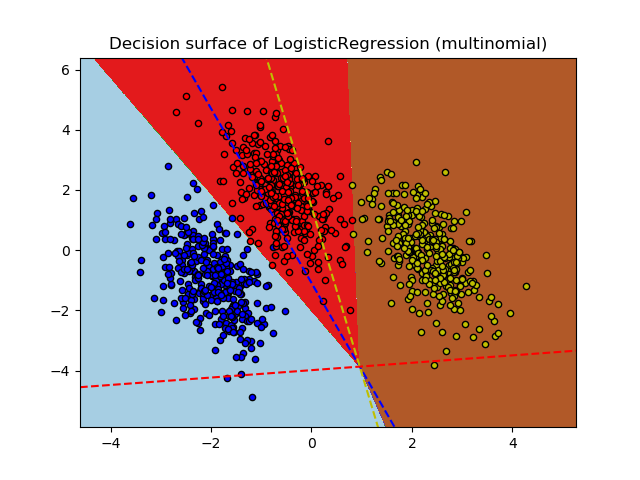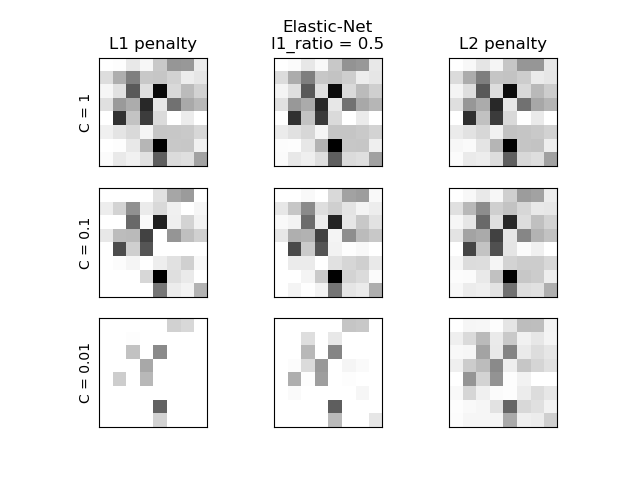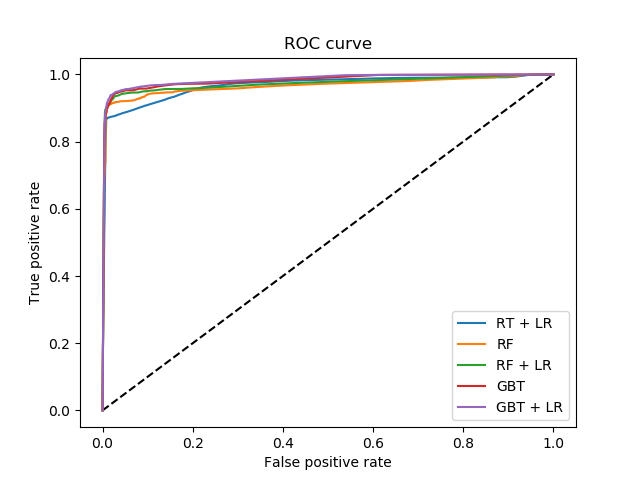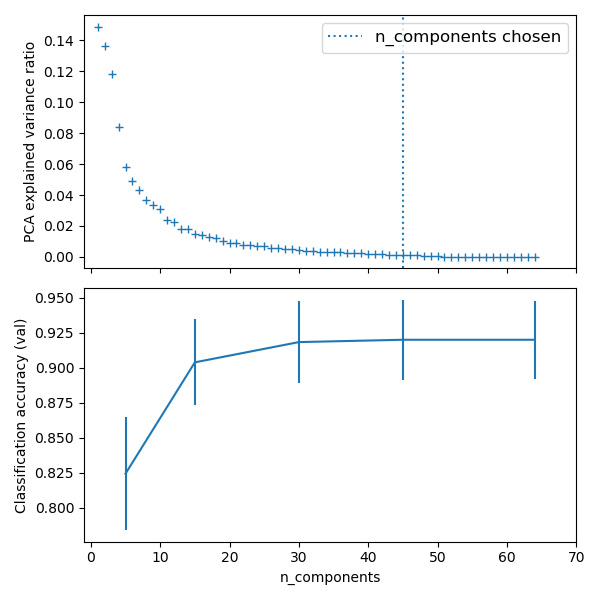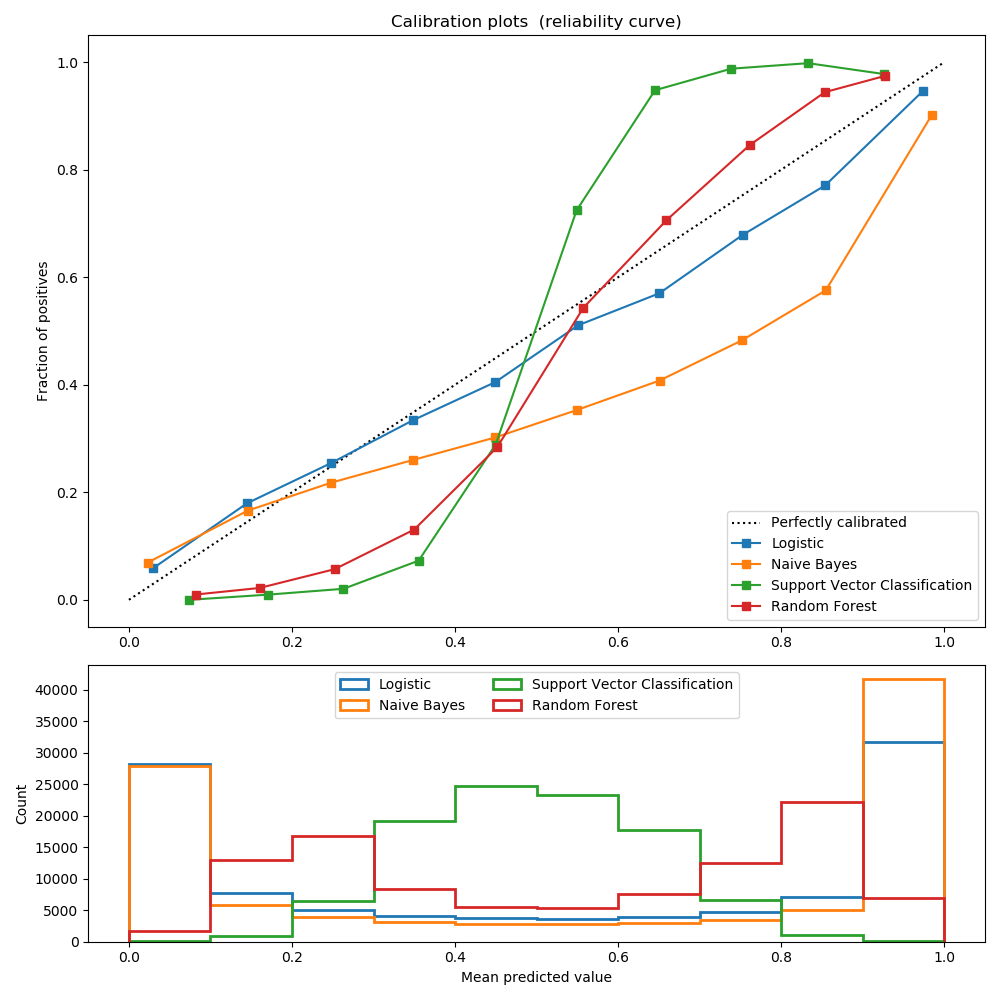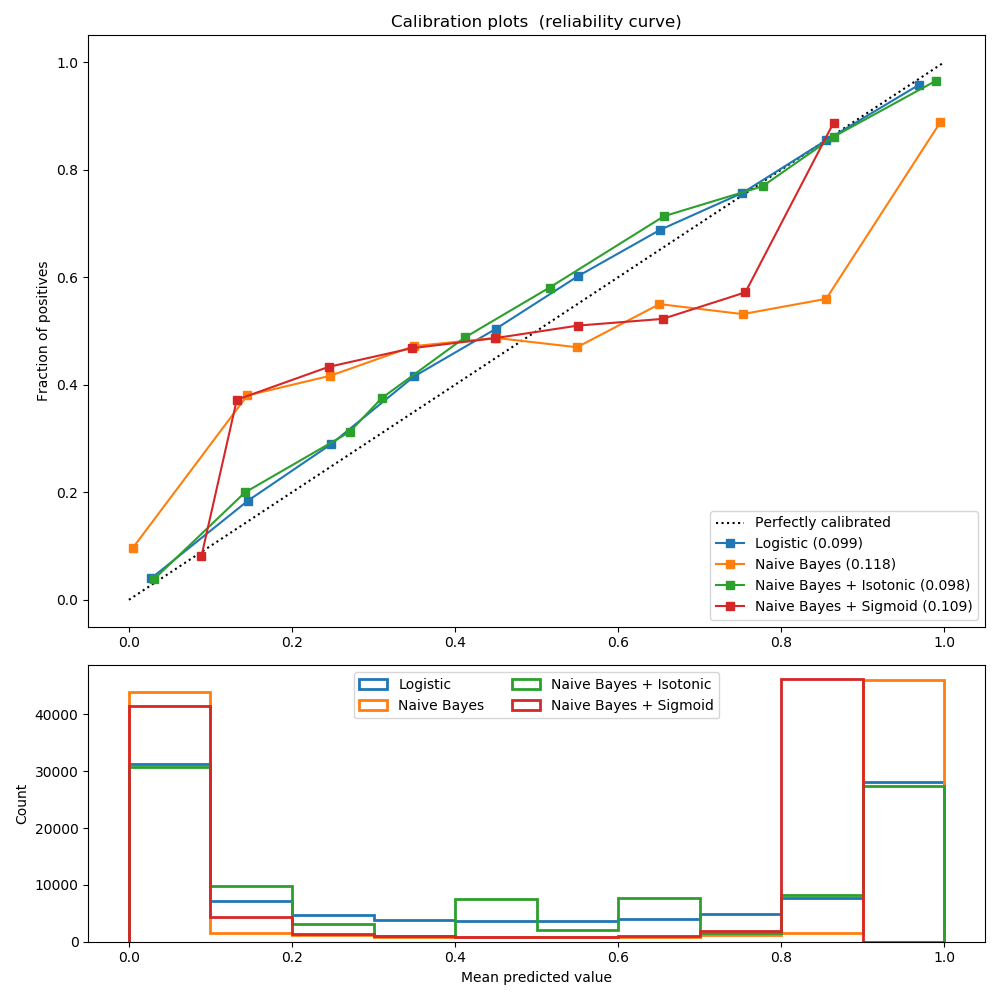# sklearn.linear_model.LogisticRegression¶

class sklearn.linear_model.LogisticRegression(penalty='l2', *, dual=False, tol=0.0001, C=1.0, fit_intercept=True, intercept_scaling=1, class_weight=None, random_state=None, solver='lbfgs', max_iter=100, multi_class='auto', verbose=0, warm_start=False, n_jobs=None, l1_ratio=None)

[源码]

Logistic回归（又名logit，MaxEnt）分类器。

“ newton-cg”，“ sag”和“ lbfgs”求解器支持带有原始公式的L2正则化或不应用正则化。“ liblinear”求解器支持L1和L2正则化，仅针对L2罚分采用双重公式。只有“ saga”求解器支持Elastic-Net正则化。

penalty {‘L1’, ‘L2’, ‘elasticnet’, ‘none’}, default=’L2’

dual bool, default=False

tol float, default=1e-4

C float, default=1.0

fit_intercept bool, default=True

intercept_scaling float, default=1

class_weight dict or ‘balanced’, default=None
{class_label: weight}的形式与类别关联的权重。如果没有给出，所有类别的权重都应该是1。

“balanced”模式使用y的值来自动调整为与输入数据中的类频率成反比的权重。如n_samples / (n_classes * np.bincount(y))

*0.17版中的新功能：*class_weight ='balanced'
random_state int, RandomState instance, default=None
solver=='sag'，'saga'或'liblinear'时，用于随机整理数据。有关详细信息，请参见词汇表
solver {‘newton-cg’, ‘lbfgs’, ‘liblinear’, ‘sag’, ‘saga’}, default=’lbfgs’

- 对于小型数据集，“ liblinear”是一个不错的选择，而对于大型数据集，“ sag”和“ saga”更快。
- 对于多类分类问题，只有'newton-cg' ，'sag'，'saga' 和 'lbfgs' 处理多项式损失。“ liblinear”仅限于“一站式”计划。
- 'newton-cg'，'lbfgs'，'sag'和'saga'处理L2或不惩罚
- 'liblinear'和'saga'也可以处理L1罚款
- “ saga”还支持“ elasticnet”惩罚
- 'liblinear'不支持设置 penalty='none'

*版本0.17中的新功能：*随机平均梯度下降求解器。

0.19版中的新功能： SAGA求解器。

max_iter int, default=100

multi_class {‘auto’, ‘ovr’, ‘multinomial’}, default=’auto’

*版本0.18中的新功能：*用于“多项式”情况的随机平均梯度下降求解器。

*在版本0.22中更改：在版本0.22中，*默认值从'ovr'更改为'auto'。
verbose int, default=0

warm_start bool, default=False

0.17版中的新功能：**warm_start支持lbfgsnewton-cgsagsaga求解器。
n_jobs int, default=None

l1_ratio float, default=None
Elastic-Net混合参数，取值范围0 <= l1_ratio <= 1。仅在penalty='elasticnet'时使用。设置l1_ratio=0等同于使用penalty='l2'，而设置l1_ratio=1等同于使用penalty='l1'。对于0 < l1_ratio <1，惩罚是L1和L2的组合。

classes_ ndarray of shape (n_classes, )

coef_ ndarray of shape (1, n_features) or (n_classes, n_features)

coef_当给定问题为二分类时，其形状为（1，n_features）。特别地，当时multi_class='multinomial'coef_对应于输出1（真），并且-coef_对应于输出0（假）。
intercept_ ndarray of shape (1,) or (n_classes,)

n_iter_ ndarray of shape (n_classes,) or (1, )

*在0.20版中更改：*在SciPy <= 1.0.0中，lbfgs迭代次数可能超过 max_itern_iter_现在输出最大的max_iter

SGDClassifier

LogisticRegressionCV

L-BFGS-B – Software for Large-scale Bound-constrained Optimization

Ciyou Zhu, Richard Byrd, Jorge Nocedal and Jose Luis Morales. http://users.iems.northwestern.edu/~nocedal/lbfgsb.html

LIBLINEAR – A Library for Large Linear Classification

https://www.csie.ntu.edu.tw/~cjlin/liblinear/

SAG – Mark Schmidt, Nicolas Le Roux, and Francis Bach

Minimizing Finite Sums with the Stochastic Average Gradient https://hal.inria.fr/hal-00860051/document

SAGA – Defazio, A., Bach F. & Lacoste-Julien S. (2014).

SAGA: A Fast Incremental Gradient Method With Support for Non-Strongly Convex Composite Objectives https://arxiv.org/abs/1407.0202

Hsiang-Fu Yu, Fang-Lan Huang, Chih-Jen Lin (2011). Dual coordinate descent

methods for logistic regression and maximum entropy models. Machine Learning 85(1-2):41-75. https://www.csie.ntu.edu.tw/~cjlin/papers/maxent_dual.pdf

>>> from sklearn.datasets import load_iris>>> from sklearn.linear_model import LogisticRegression>>> X, y = load_iris(return_X_y=True)>>> clf = LogisticRegression(random_state=0).fit(X, y)>>> clf.predict(X[:2, :])array([0, 0])>>> clf.predict_proba(X[:2, :])array([[9.8...e-01, 1.8...e-02, 1.4...e-08],       [9.7...e-01, 2.8...e-02, ...e-08]])>>> clf.score(X, y)0.97...

decision_function(self, X) 预测样本的置信度得分。
densify(self) 将系数矩阵转换为密集数组格式。
fit(self, X, y[, sample_weight]) 根据给定的训练数据拟合模型。
get_params(self[, deep]) 获取此估计器的参数。
predict(self, X) 预测X中样本的类别标签。
predict_log_proba(self, X) 预测概率估计的对数。
predict_proba(self, X) 概率估计。
score(self, X, y[, sample_weight]) 返回给定测试数据和标签上的平均准确度。
set_params(self, **params) 设置此估计器的参数。
sparsify(self) 将系数矩阵转换为稀疏格式。
__init__(self, penalty='l2', *, dual=False, tol=0.0001, C=1.0, fit_intercept=True, intercept_scaling=1, class_weight=None, random_state=None, solver='lbfgs', max_iter=100, multi_class='auto', verbose=0, warm_start=False, n_jobs=None, l1_ratio=None)

[源码]

decision_function(self, X)

[源码]

X array_like or sparse matrix, shape (n_samples, n_features)

array, shape=(n_samples,) if n_classes == 2 else (n_samples, n_classes)

densify(self)

[源码]

coef_数值（返回）转换为numpy.ndarray。这是coef_的默认格式，并且是拟合模型所需的格式，因此仅在之前被稀疏化的模型上才需要调用此方法。否则，它是无操作的。

self 拟合估计器。
fit（self，X，y，sample_weight = None ）

[源码]

X {array-like, sparse matrix} of shape (n_samples, n_features)

y array-like of shape (n_samples,)

sample_weight array-like of shape (n_samples,) default=None

0.17版中的新功能：sample_weight支持LogisticRegression

self 拟合估计器。

SAGA求解器支持float64和float32位数组。

get_params（self，deep = True ）

[源码]

deep bool, default=True

params mapping of string to any

predict(self, X)

[源码]

X array_like or sparse matrix, shape (n_samples, n_features)

C array, shape [n_samples]

predict_log_proba(self, X)

[源码]

X array-like of shape (n_samples, n_features)

T array-like of shape (n_samples, n_classes)

predict_proba(self, X)

[源码]

X array-like of shape (n_samples, n_features)

T array-like of shape (n_samples, n_classes)

score(self, X, y, sample_weight=None)

[源码]

X array-like of shape (n_samples, n_features)

y array-like of shape (n_samples,) or (n_samples, n_outputs)
X的真实标签。
sample_weight array-like of shape (n_samples,), default=None

score float

set_params(self, **params)

[源码]

**params dict

self object

sparsify(self)

[源码]

coef_数值转换为scipy.sparse矩阵，对于L1正规化的模型，该矩阵比通常的numpy.ndarray具有更高的内存和存储效率。

intercept_数值未转换。

self 拟合估计器。

## sklearn.linear_model.LogisticRegression使用示例¶